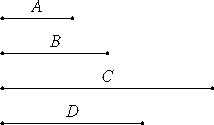# Proposition 1

If two similar plane numbers multiplied by one another make some number, then the product is square.

Let A and B be two similar plane numbers, and let A multiplied by B make C.

I say that C is square.

Multiply A by itself to make D. Then D is square.VII.17

Since then A multiplied by itself makes D, and multiplied by B makes C, therefore A is to B as D is to C.

VIII.18

And, since A and B are similar plane numbers, therefore one mean proportional number falls between A and B.

VIII.8

Since as many number fall in continued proportion between those which have the same ratio, therefore one mean proportional number falls between D and C also.

VIII.22

And D is square, therefore C is also square.

Therefore, if two similar plane numbers multiplied by one another make some number, then the product is square.

Q.E.D.

## Guide

Although this is the first proposition in Book IX, it and the succeeding propositions continue those of Book VIII without break.

To illustrate this proposition, consider the two similar plane numbers a = 18 and b = 8, as illustrated in the Guide to VII.Def.21. According to VIII.18, there is a mean proportional between them, namely, 12. And the square of the mean proportional is their product, ab = 144.

#### Outline of the proof

It is not clear why the proof of the proposition does not use the fact that the square of the mean proportional of two numbers equals their product, but instead uses slightly more complicated reasoning.

Let a and b be the given similar plane numbers. Then there is a mean proportional between them (VIII.18). And, since a : b = a2:ab, therefore there is also a mean proportional between a2 and ab (VIII.1). But since a2 is a square, therefore ab is also a square (VIII.22). Thus, the product of the original similar plane numbers is a square.

#### Use of this proposition

The next proposition IX.2 is the converse of this one. This proposition is used in X.29.